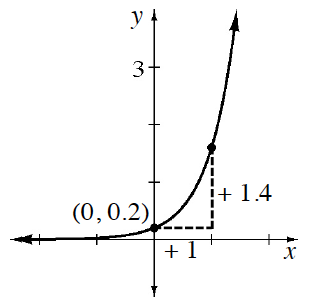### Home > INT1 > Chapter 9 > Lesson 9.2.1 > Problem9-48

9-48.

Use the graph below to write an exponential equation in $f(x) = ab^x$ form.

What part of the equation, $y = ab^{x}$, can be easily calculated knowing the point $(0, 0.2)$?

The second point is $(1, 1.6)$. Use this point to calculate $b$.